# Exam-Style Questions.

## Problems adapted from questions set for previous Mathematics exams.

### 1.

GCSE Higher

The grouped frequency table gives information about the times, in minutes, that 90 commuters take to get home from work.

Time (t minutes)Frequency
$$0 \lt t \le 10$$6
$$10 \lt t \le 20$$32
$$20 \lt t \le 30$$22
$$30 \lt t \le 40$$10
$$40 \lt t \le 50$$10
$$50 \lt t \le 60$$7
$$60 \lt t \le 70$$3

(a) Complete the cumulative frequency table.

Time (t minutes)Cumulative frequency
$$0 \lt t \le 10$$
$$0 \lt t \le 20$$
$$0 \lt t \le 30$$
$$0 \lt t \le 40$$
$$0 \lt t \le 50$$
$$0 \lt t \le 60$$
$$0 \lt t \le 70$$

(b) On the grid, draw the cumulative frequency graph for this information.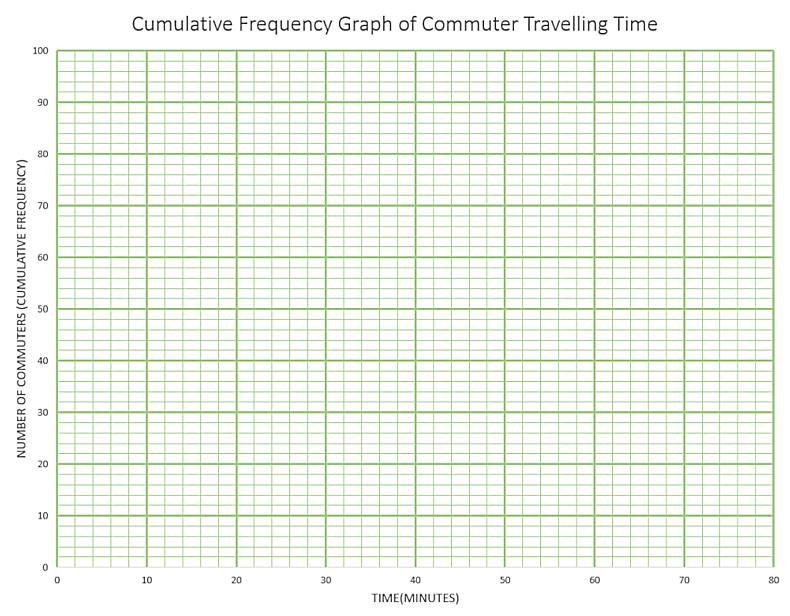(c) Use your graph to find an estimate for the percentage of these commuters who take more than 45 minutes to get home from work.

### 2.

GCSE Higher

The table shows information about the heights of 85 stalagmites.

Height ($$h$$ cm) Frequency
$$10\lt h \le 15$$9
$$15\lt h \le 20$$13
$$20\lt h \le 25$$18
$$25\lt h \le 30$$22
$$30\lt h \le 35$$15
$$35\lt h \le 40$$8

(a) Find the class interval that contains the median.

(b) On the grid below, draw a frequency polygon for the information in the table.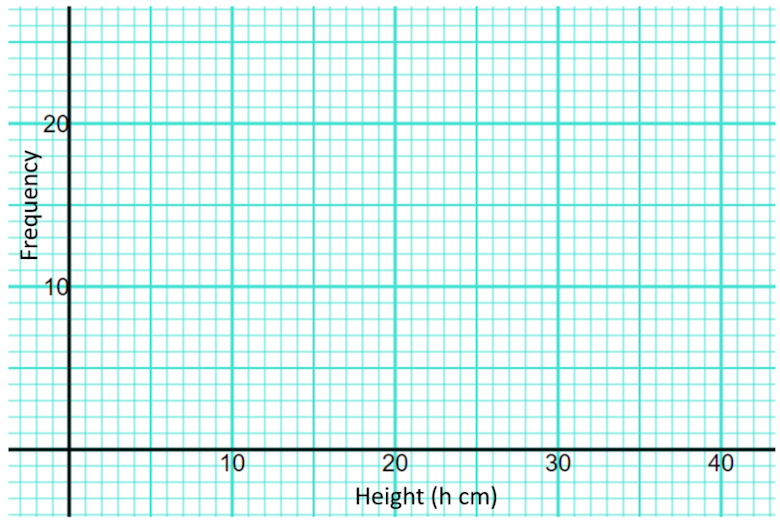### 3.

IGCSE Extended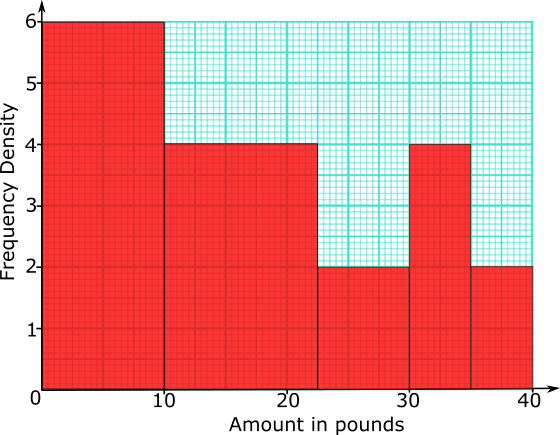155 people were asked how much money they would pay for particular three-course meal in a special restaurant.

The histogram shows the results of the survey.

(a) Complete the frequency table for information shown in the histogram.

Amount (£$$x$$) Frequency $$0\lt x \le 10$$ 20

(b) Use your frequency table to calculate an estimate of the mean amount these people would pay for the meal.

### 4.

GCSE Higher

The scatter graph gives information about the marks earned in a Statistic exam and a Mathematics exam by each of 13 students.The box plot for the Mathematics exam for the 13 students is drawn on the grid below.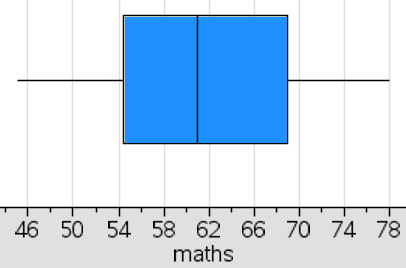(a) Draw the box plot for the Statistics exam.

(b) Compare the distributions of marks scored in the two exams.

### 5.

GCSE Higher

Here is some information about the length of time children spent in a swimming pool at a holiday resort during a heat wave.

• Shortest time 30 minutes
• Longest time 5 hours
• Lower quartile 90 minutes
• Interquartile range 2 hours
• Median time 2 hours 30 minutes

Draw a box plot to show this information.

### 6.

GCSE Higher

The table shows the marks earned by 200 students taking a Maths exam.

 Mark (n) $$0\lt n \le 10$$ $$10\lt n \le 20$$ $$20\lt n \le 30$$ $$30\lt n \le 40$$ $$40\lt n \le 50$$ $$50\lt n \le 60$$ $$60\lt n \le 70$$ $$70\lt n \le 80$$ Frequency 3 7 33 42 54 35 20 6

(a) Use the data in the table above to complete the following cumulative frequency table

 Mark (n) $$n \le 10$$ $$n \le 20$$ $$n \le 30$$ $$n \le 40$$ $$n \le 50$$ $$n \le 60$$ $$n \le 70$$ $$n \le 80$$ Cumulative Frequency 200

(b) Draw the cumulative frequency curve on graph paper.

(c) Use your graph to estimate the lowest mark that B grade will be awarded for.

### 7.

GCSE Higher

The table shows the amount of time, in months, taken to sell houses on the Happyland housing estate.

 Time (m months) Frequency 0 < m ≤ 2 10 2 < m ≤ 5 21 5 < m ≤ 10 25 10 < m ≤ 15 20 15 < m ≤ 20 25

(a) Draw a histogram for the information in the table.

(b) Find an estimate for the median.

### 8.

IB StudiesThe distribution of daily average wind speed on an island over a period of 120 days is displayed on this box-and-whisker diagram.

(a) Write down the median wind speed.

(b) Write down the minimum wind speed.

(c) Find the interquartile range.

(d) Write down the number of days the wind speed was between 20 kmh-1 and 51 kmh-1.

(e) Write down the number of days the wind speed was between 9 kmh-1 and 68 kmh-1.

### 9.

IB Studies

The following grouped frequency table shows the length of time, $$t$$, in minutes, visitors watched an octopus swimming around a tank at an aquarium.

Time ($$t$$)Visitors
$$0\lt t \le 5$$23
$$5\lt t \le 10$$13
$$10\lt t \le 15$$9
$$15\lt t \le 20$$6
$$20\lt t \le 25$$2
$$25\lt t \le 30$$1

(a) Write down the total number of visitors who were included in the survey.

(b) Write down the mid-interval value for the $$20\lt t \le 25$$ group.

(c) Find an estimate of the mean time visitors took watching the octopus.

The information above has been rewritten as a cumulative frequency table.

 Time ($$t$$) Cumulative frequency $$t \le 5$$ $$t \le 10$$ $$t \le 15$$ $$t \le 20$$ $$t \le 25$$ $$t \le 30$$ 23 36 $$a$$ 51 53 $$b$$

(d) Write down the values of $$a$$ and $$b$$.

This information is shown in the following cumulative frequency graph.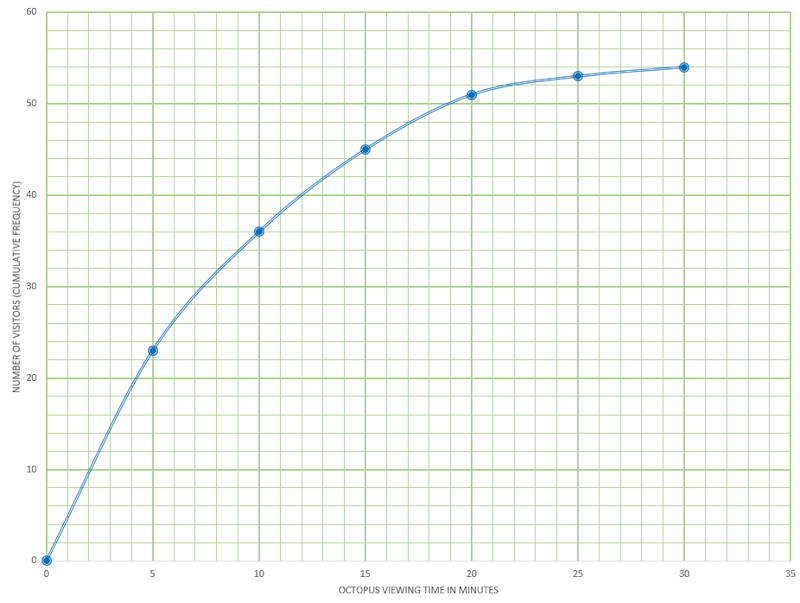(e) Use the graph to estimate the maximum time taken watching the octopus for the first 32 visitors (arranged in order of increasing viewing time).

(f) Use the graph to estimate the number of visitors who spent less than 13 minutes watching the octopus.

(g) Use the graph to estimate the number of visitors who take more than 17 minutes watching the octopus.

### 10.

IB Applications and Interpretation

Derek has put together a fantasy football squad and has recorded the heights of all the players in the squad rounded to the nearest centimetre. The data is illustrated in the following box and whisker diagram.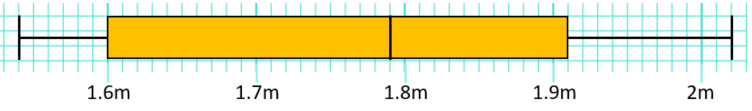(a) Write down the median height of the players.

(b) Write down the upper quartile height.

(c) Find the interquartile range of the heights.

The height of these players are normally distributed.

(d) Find the height of the tallest possible player that is not an outlier. Give your answer to the nearest centimetre.

### 11.

GCSE Higher

The charts below show the results of pupils in a Year Group answering twenty mental arithmetic questions.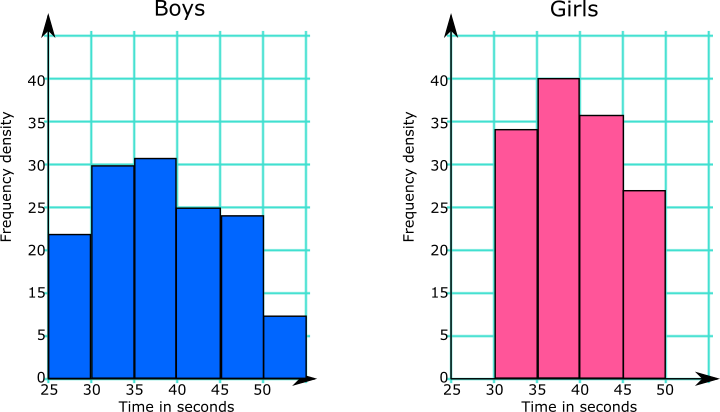What information from the diagrams can be used to support or counter each of these statements?

(a) Girls are better at mental arithmetic than boys.

(b) The range of the boys' times is greater than the range of the girls' times.

The Head of the school would like to use this data to make statements about all of the pupils in the school.

(c) Comment on whether any conclusions can be drawn for the whole school population from the results of this Year Group.

### 12.

GCSE Higher

Poppy Pringle recorded the heights in centimetres of the sunflowers growing in her brother's and sister's gardens.Here are the heights of the 19 sunflowers in her brother's garden:

$$94, 103, 110, 111, 123, 143, 150, 150, 151, 156, 157, 160, 170, 182, 201, 220, 250, 255, 231$$

 Median Lower quartile Upper quartile Shortest Tallest

Here is some information about the sunflowers in Poppy's sister's garden:

 Median 160 Lower quartile 143 Upper quartile 172 Shortest 110 Tallest 199

Poppy says that the sunflowers in her brother's garden are shorter that those in her sister's garden.

(b) Is Poppy correct?

Poppy says that the sunflowers in her brother's garden vary more in height than those in her sister's garden.

(c) Is Poppy correct?

### 13.

IB Analysis and Approaches

A TV company surveyed 88 of its employees to find out how much time they spend travelling to work on a given day. The results of the survey are shown in the following cumulative frequency diagram.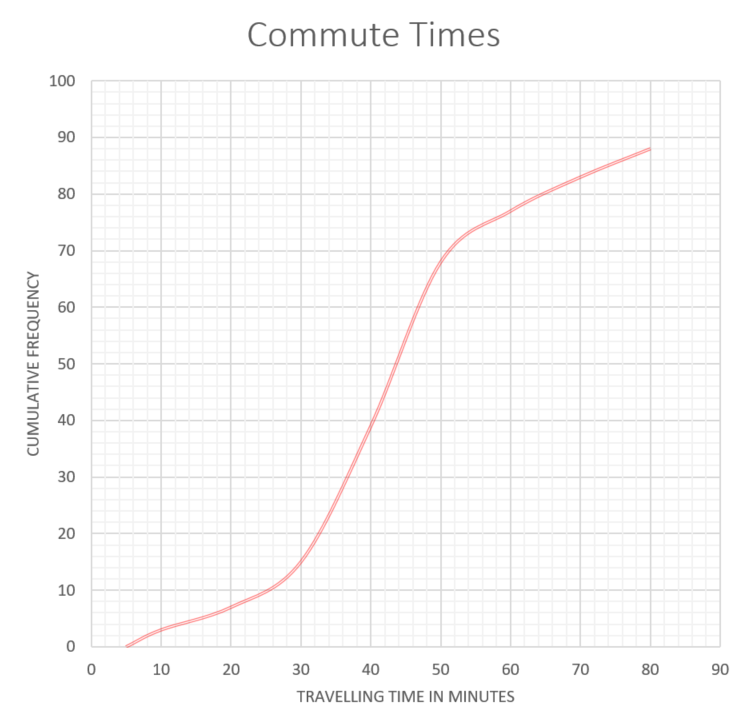(a) Find the median number of minutes spent travelling to work.

(b) Find the interquartile range.

(c) Find the number of employees whose travelling time is within 20 minutes of the median.

(d) Only 10% of the employees spent less than k minutes travelling to work. Find the value of k.

The results of the survey can also be displayed on the following box-and-whisker diagram.(e) Write down the value of a.

(f) Find the value of b.

(g) Travelling times of less than p minutes are considered outliers. Find the value of p .

### 14.

IB Studies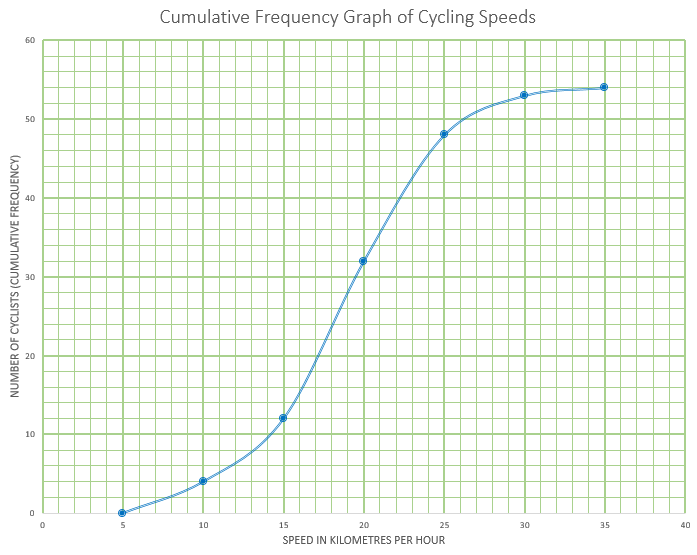This cumulative frequency graph shows the speeds in kmh-1 of cyclists passing a certain point on a race track.

(a) Estimate the minimum possible speed of one of these cyclists.

(b) Find the median speed of the cyclists.

(c) Write down the 65th percentile.

(d) Calculate the interquartile range.

(e) Find the number of these cyclists that were travelling faster than 22 kmh-1

The table shows the speeds of these cyclists.

Speed of Cyclists (s)Number of Cyclists
$$0 \lt s \le 5$$0
$$5 \lt s \le 10$$$$a$$
$$10 \lt s \le 15$$8
$$15 \lt s \le 20$$20
$$20 \lt s \le 25$$16
$$25 \lt s \le 30$$5
$$30 \lt s \le 35$$$$b$$

(f) Find the value of $$a$$ and of $$b$$

(g) Write down the modal class.

(h) Write down the mid-interval value for the modal class.

(i) Use your graphic display calculator to calculate an estimate of the mean speed of these cyclists.

(j) Use your graphic display calculator to calculate an estimate of the standard deviation of the speeds of these cyclists.

If you would like space on the right of the question to write out the solution try this Thinning Feature. It will collapse the text into the left half of your screen but large diagrams will remain unchanged.

The exam-style questions appearing on this site are based on those set in previous examinations (or sample assessment papers for future examinations) by the major examination boards. The wording, diagrams and figures used in these questions have been changed from the originals so that students can have fresh, relevant problem solving practice even if they have previously worked through the related exam paper.

The solutions to the questions on this website are only available to those who have a Transum Subscription.

Exam-Style Questions Main Page

Search for exam-style questions containing a particular word or phrase:

To search the entire Transum website use the search box in the grey area below.